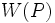# Characteristic p-functor that gives a characteristic subgroup

(diff) ← Older revision | Latest revision (diff) | Newer revision → (diff)
This article defines a property that can be evaluated for a characteristic p-functor in the context of a finite group.|View other such properties

## Definition

Suppose$p$ is a prime number and$G$ is a finite group such that$W$ is a conjugacy functor for$G$ for the prime$p$ arising from a characteristic p-functor. We say that$W$ is a characteristic p-functor that gives a characteristic subgroup if it satisfies the following equivalent conditions:

1. For every pair of$p$-Sylow subgroups$P,Q$ of$G$,$W(P) = W(Q)$.
2. For every pair of$p$-Sylow subgroups$P,Q$ of$G$,$W(P)$ is a normal subgroup of$Q$.
3. Each of these:
•$W$ is a weakly closed conjugacy functor and there exists a$p$-Sylow subgroup$P$ of$G$ such that$W(P) \le O_p(G)$ where$O_p(G)$ is the p-core of$G$.
•$W$ is a weakly closed conjugacy functor and for every$p$-Sylow subgroup$P$ of$G$,$W(P) \le O_p(G)$ where$O_p(G)$ is the$p$-core of$G$.
4. Each of these:
• There exists a$p$-Sylow subgroup$P$ of$G$ such that$W(P)$ is a characteristic subgroup of$G$.
• For every$p$-Sylow subgroup$P$ of$G$,$W(P)$ is a characteristic subgroup of$G$.
5. Each of these:
• There exists a$p$-Sylow subgroup$P$ of$G$ such that$W(P)$ is a normal subgroup of$G$.
• For every$p$-Sylow subgroup$P$ of$G$,$W(P)$ is a normal subgroup of$G$.

### Equivalence of definitions

Further information: equivalence of normality and characteristicity conditions for conjugacy functor